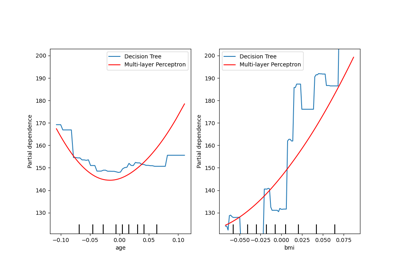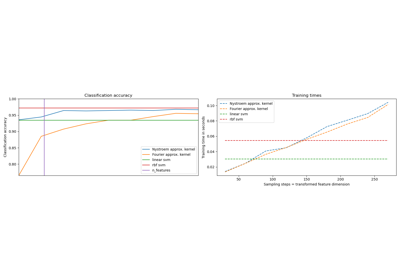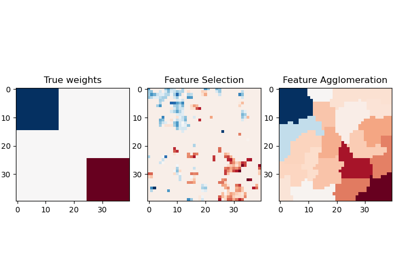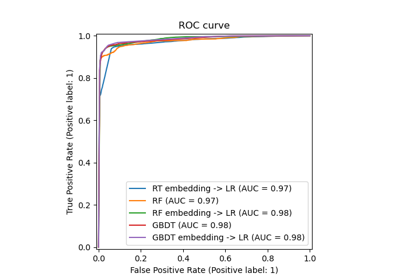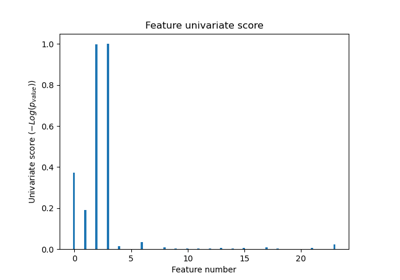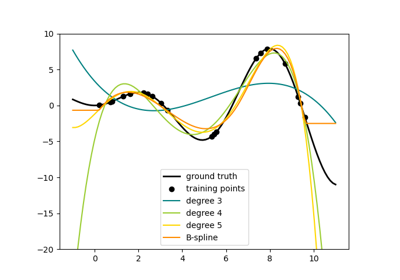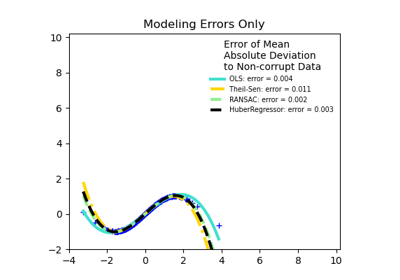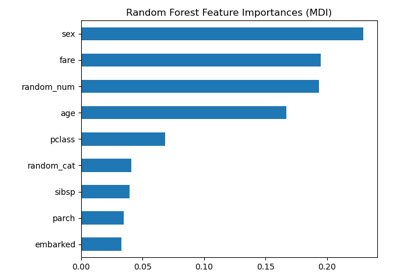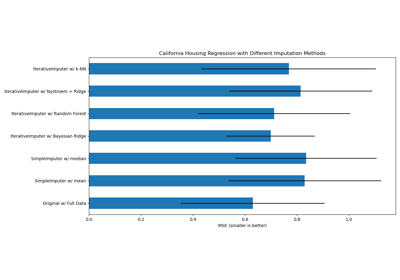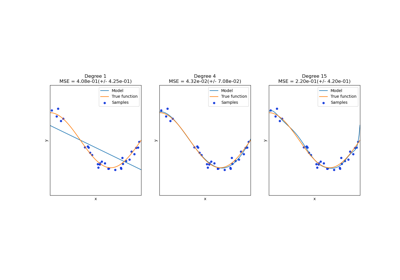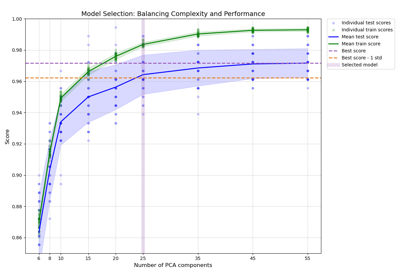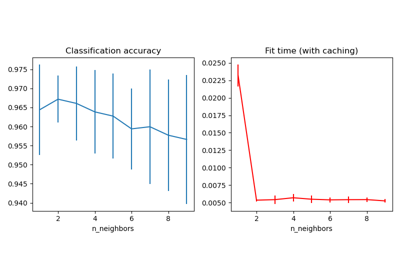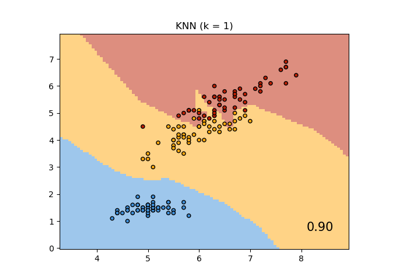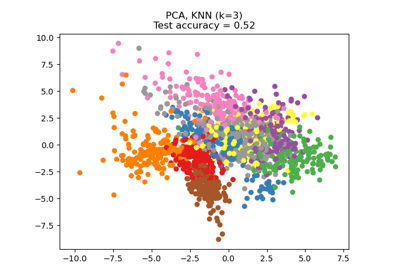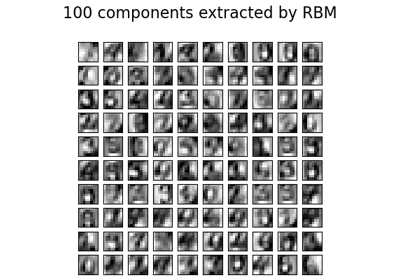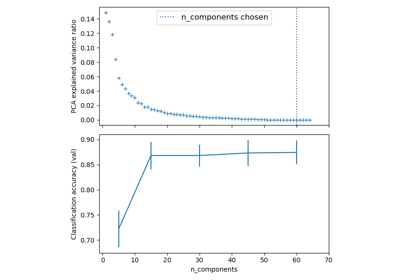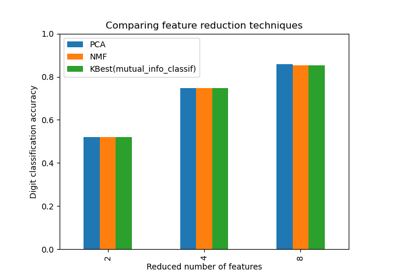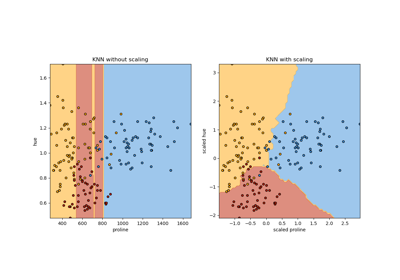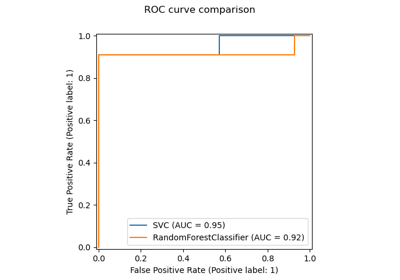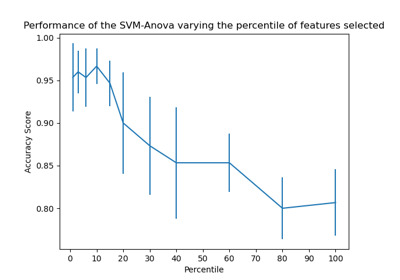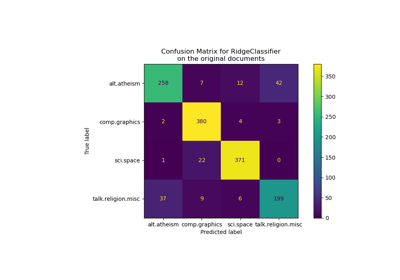# sklearn.pipeline.Pipeline¶

class sklearn.pipeline.Pipeline(steps, memory=None, verbose=False)[source]

Pipeline of transforms with a final estimator.

Sequentially apply a list of transforms and a final estimator. Intermediate steps of the pipeline must be ‘transforms’, that is, they must implement fit and transform methods. The final estimator only needs to implement fit. The transformers in the pipeline can be cached using memory argument.

The purpose of the pipeline is to assemble several steps that can be cross-validated together while setting different parameters. For this, it enables setting parameters of the various steps using their names and the parameter name separated by a ‘__’, as in the example below. A step’s estimator may be replaced entirely by setting the parameter with its name to another estimator, or a transformer removed by setting it to ‘passthrough’ or None.

Read more in the User Guide.

Parameters
stepslist

List of (name, transform) tuples (implementing fit/transform) that are chained, in the order in which they are chained, with the last object an estimator.

memoryNone, str or object with the joblib.Memory interface, optional

Used to cache the fitted transformers of the pipeline. By default, no caching is performed. If a string is given, it is the path to the caching directory. Enabling caching triggers a clone of the transformers before fitting. Therefore, the transformer instance given to the pipeline cannot be inspected directly. Use the attribute named_steps or steps to inspect estimators within the pipeline. Caching the transformers is advantageous when fitting is time consuming.

verbosebool, default=False

If True, the time elapsed while fitting each step will be printed as it is completed.

Attributes
named_stepsbunch object, a dictionary with attribute access

Read-only attribute to access any step parameter by user given name. Keys are step names and values are steps parameters.

sklearn.pipeline.make_pipeline

Convenience function for simplified pipeline construction.

Examples

>>> from sklearn import svm
>>> from sklearn.datasets import make_classification
>>> from sklearn.feature_selection import SelectKBest
>>> from sklearn.feature_selection import f_regression
>>> from sklearn.pipeline import Pipeline
>>> # generate some data to play with
>>> X, y = make_classification(
...     n_informative=5, n_redundant=0, random_state=42)
>>> # ANOVA SVM-C
>>> anova_filter = SelectKBest(f_regression, k=5)
>>> clf = svm.SVC(kernel='linear')
>>> anova_svm = Pipeline([('anova', anova_filter), ('svc', clf)])
>>> # You can set the parameters using the names issued
>>> # For instance, fit using a k of 10 in the SelectKBest
>>> # and a parameter 'C' of the svm
>>> anova_svm.set_params(anova__k=10, svc__C=.1).fit(X, y)
Pipeline(steps=[('anova', SelectKBest(...)), ('svc', SVC(...))])
>>> prediction = anova_svm.predict(X)
>>> anova_svm.score(X, y)
0.83
>>> # getting the selected features chosen by anova_filter
>>> anova_svm['anova'].get_support()
array([False, False,  True,  True, False, False,  True,  True, False,
True, False,  True,  True, False,  True, False,  True,  True,
False, False])
>>> # Another way to get selected features chosen by anova_filter
>>> anova_svm.named_steps.anova.get_support()
array([False, False,  True,  True, False, False,  True,  True, False,
True, False,  True,  True, False,  True, False,  True,  True,
False, False])
>>> # Indexing can also be used to extract a sub-pipeline.
>>> sub_pipeline = anova_svm[:1]
>>> sub_pipeline
Pipeline(steps=[('anova', SelectKBest(...))])
>>> coef = anova_svm[-1].coef_
>>> anova_svm['svc'] is anova_svm[-1]
True
>>> coef.shape
(1, 10)
>>> sub_pipeline.inverse_transform(coef).shape
(1, 20)


Methods

 decision_function(self, X) Apply transforms, and decision_function of the final estimator fit(self, X[, y]) Fit the model fit_predict(self, X[, y]) Applies fit_predict of last step in pipeline after transforms. fit_transform(self, X[, y]) Fit the model and transform with the final estimator get_params(self[, deep]) Get parameters for this estimator. predict(self, X, \*\*predict_params) Apply transforms to the data, and predict with the final estimator predict_log_proba(self, X) Apply transforms, and predict_log_proba of the final estimator predict_proba(self, X) Apply transforms, and predict_proba of the final estimator score(self, X[, y, sample_weight]) Apply transforms, and score with the final estimator score_samples(self, X) Apply transforms, and score_samples of the final estimator. set_params(self, \*\*kwargs) Set the parameters of this estimator.
__init__(self, steps, memory=None, verbose=False)[source]

Initialize self. See help(type(self)) for accurate signature.

decision_function(self, X)[source]

Apply transforms, and decision_function of the final estimator

Parameters
Xiterable

Data to predict on. Must fulfill input requirements of first step of the pipeline.

Returns
y_scorearray-like of shape (n_samples, n_classes)
fit(self, X, y=None, **fit_params)[source]

Fit the model

Fit all the transforms one after the other and transform the data, then fit the transformed data using the final estimator.

Parameters
Xiterable

Training data. Must fulfill input requirements of first step of the pipeline.

yiterable, default=None

Training targets. Must fulfill label requirements for all steps of the pipeline.

**fit_paramsdict of string -> object

Parameters passed to the fit method of each step, where each parameter name is prefixed such that parameter p for step s has key s__p.

Returns
selfPipeline

This estimator

fit_predict(self, X, y=None, **fit_params)[source]

Applies fit_predict of last step in pipeline after transforms.

Applies fit_transforms of a pipeline to the data, followed by the fit_predict method of the final estimator in the pipeline. Valid only if the final estimator implements fit_predict.

Parameters
Xiterable

Training data. Must fulfill input requirements of first step of the pipeline.

yiterable, default=None

Training targets. Must fulfill label requirements for all steps of the pipeline.

**fit_paramsdict of string -> object

Parameters passed to the fit method of each step, where each parameter name is prefixed such that parameter p for step s has key s__p.

Returns
y_predarray-like
fit_transform(self, X, y=None, **fit_params)[source]

Fit the model and transform with the final estimator

Fits all the transforms one after the other and transforms the data, then uses fit_transform on transformed data with the final estimator.

Parameters
Xiterable

Training data. Must fulfill input requirements of first step of the pipeline.

yiterable, default=None

Training targets. Must fulfill label requirements for all steps of the pipeline.

**fit_paramsdict of string -> object

Parameters passed to the fit method of each step, where each parameter name is prefixed such that parameter p for step s has key s__p.

Returns
Xtarray-like of shape (n_samples, n_transformed_features)

Transformed samples

get_params(self, deep=True)[source]

Get parameters for this estimator.

Parameters
deepboolean, optional

If True, will return the parameters for this estimator and contained subobjects that are estimators.

Returns
paramsmapping of string to any

Parameter names mapped to their values.

property inverse_transform

Apply inverse transformations in reverse order

All estimators in the pipeline must support inverse_transform.

Parameters
Xtarray-like of shape (n_samples, n_transformed_features)

Data samples, where n_samples is the number of samples and n_features is the number of features. Must fulfill input requirements of last step of pipeline’s inverse_transform method.

Returns
Xtarray-like of shape (n_samples, n_features)
predict(self, X, **predict_params)[source]

Apply transforms to the data, and predict with the final estimator

Parameters
Xiterable

Data to predict on. Must fulfill input requirements of first step of the pipeline.

**predict_paramsdict of string -> object

Parameters to the predict called at the end of all transformations in the pipeline. Note that while this may be used to return uncertainties from some models with return_std or return_cov, uncertainties that are generated by the transformations in the pipeline are not propagated to the final estimator.

Returns
y_predarray-like
predict_log_proba(self, X)[source]

Apply transforms, and predict_log_proba of the final estimator

Parameters
Xiterable

Data to predict on. Must fulfill input requirements of first step of the pipeline.

Returns
y_scorearray-like of shape (n_samples, n_classes)
predict_proba(self, X)[source]

Apply transforms, and predict_proba of the final estimator

Parameters
Xiterable

Data to predict on. Must fulfill input requirements of first step of the pipeline.

Returns
y_probaarray-like of shape (n_samples, n_classes)
score(self, X, y=None, sample_weight=None)[source]

Apply transforms, and score with the final estimator

Parameters
Xiterable

Data to predict on. Must fulfill input requirements of first step of the pipeline.

yiterable, default=None

Targets used for scoring. Must fulfill label requirements for all steps of the pipeline.

sample_weightarray-like, default=None

If not None, this argument is passed as sample_weight keyword argument to the score method of the final estimator.

Returns
scorefloat
score_samples(self, X)[source]

Apply transforms, and score_samples of the final estimator.

Parameters
Xiterable

Data to predict on. Must fulfill input requirements of first step of the pipeline.

Returns
y_scorendarray, shape (n_samples,)
set_params(self, **kwargs)[source]

Set the parameters of this estimator.

Valid parameter keys can be listed with get_params().

Returns
self
property transform

Apply transforms, and transform with the final estimator

This also works where final estimator is None: all prior transformations are applied.

Parameters
Xiterable

Data to transform. Must fulfill input requirements of first step of the pipeline.

Returns
Xtarray-like of shape (n_samples, n_transformed_features)

## Examples using sklearn.pipeline.Pipeline¶